# I like to MVO it!

In our last post, we ran through a bunch of weighting scenarios using our returns simulation. This resulted in three million portfolios comprised in part, or total, of four assets: stocks, bonds, gold, and real estate. These simulations relaxed the allocation constraints to allow us to exclude assets, yielding a wider range of return and risk results, while lowering the likelihood of achieving our risk and return targets. We bucketed the portfolios to simplify the analysis around the risk-return trade off. We then calculated the median returns and risk for each bucket and found that some buckets achieved Sharpe ratios close to or better than that implied by our original risk-return constraint. Cutting the data further, we calculated the average weights for the better Sharpe ratio portfolios. The result: relatively equal-weighting tended to produce a better risk-reward outcome than significant overweighting.

At the end of the post we noted that we could have a bypassed much of this data wrangling and simply calculated the optimal portfolio weights for various risk profiles using mean-variance optimization. That is what we plan to do today.

The madness behind all this data wrangling was to identify the best return afforded by a given level of risk. Mean-variance optimization (MVO) solves that problem more elegantly than our “hacky” methods. It uses quadratic programming1 to minimize the portfolio variance by altering the weights of the various assets in the portfolio. It is subject to the constraints (in the simplest form) that the return of any particular portfolio is at least equal to the expected return of the portfolio2 and the weights of the assets sum to one.

More formally it can be expressed as follows:

Minimize: $$\frac{1}{2}w'\sum\ w$$
Subject to: $$r'w = \mu\ and\ e'w = 1$$

Here $$w$$ = asset weights, $$\sum$$ = the covariance matrix of the assets with themselves and every other asset, $$r$$ = returns of the assets, $$\mu$$ = expected return of the portfolio, $$e'$$ = a vector of ones. It is understood that one is employing matrix notation, so the $$w'$$ is the transpose of $$w$$.

If you understand that, it’s probably the roughest rendition of MVO you’ve seen and if you don’t, don’t worry about it. The point is through some nifty math, you can solve for the precise weights so that every portfolio that falls along a line has the lowest volatility for a given level of return or the highest return for a given level of volatility. This line is called the efficient frontier since efficiency in econospeak means every asset is optimally allocated and frontier, well you get that one we hope.

What does this look like in practice? Let’s bring back our original portfolio, run the simulations, and then calculate the efficient frontier. We graph our original simulation with the original weighting constraint (all assets are in the portfolio) below.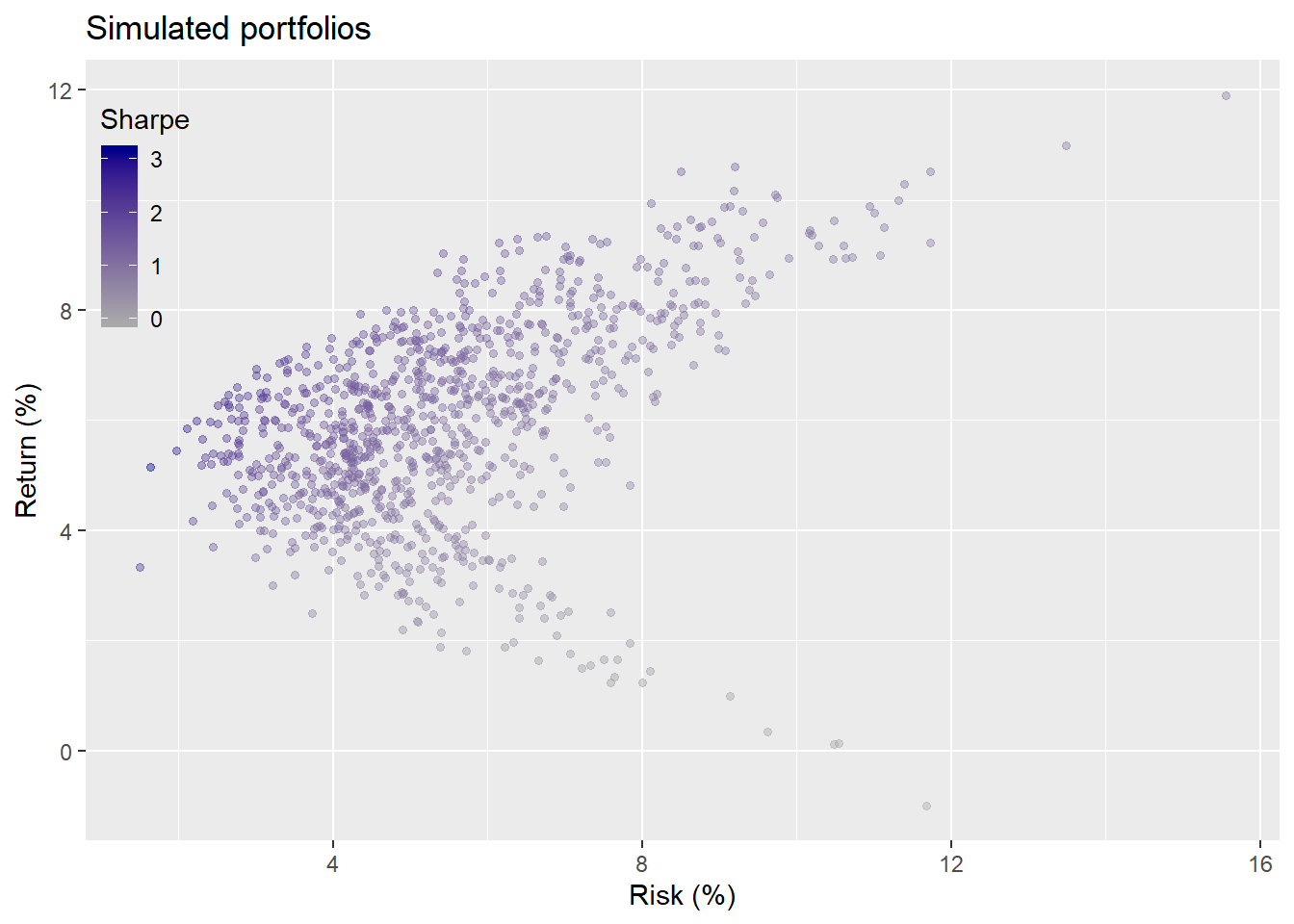Recall that after we ran this simulation we averaged the weightings for those portfolios that achieved our constraints of not less than a 7% return and not more 10% risk on an annual basis. We then applied that weighting to our first five year test period. We show the weighting below.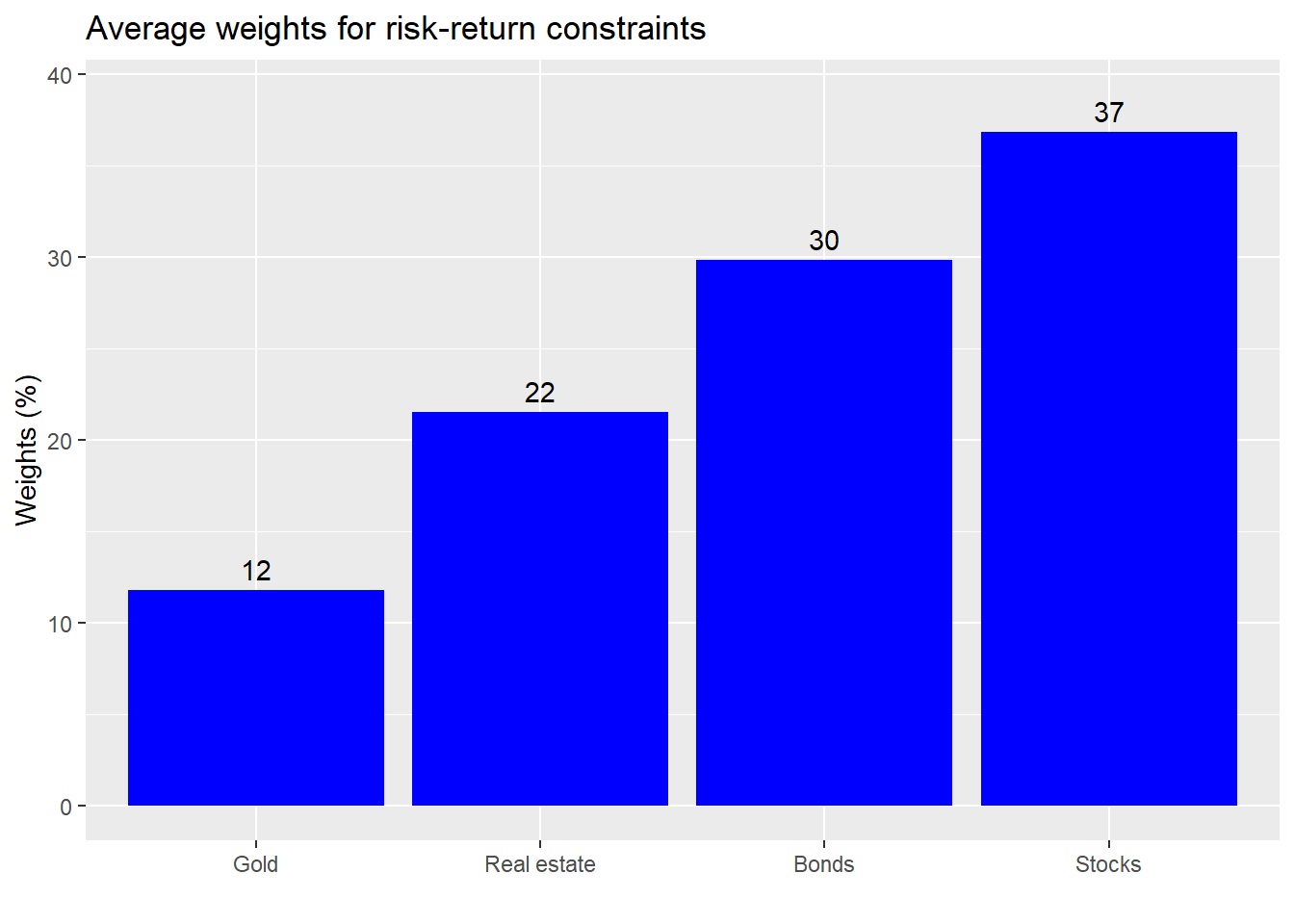Before we look at the forward returns and the efficient frontier, let’s see where our portfolio lies in the original simulation to orient ourselves. It’s the red dot.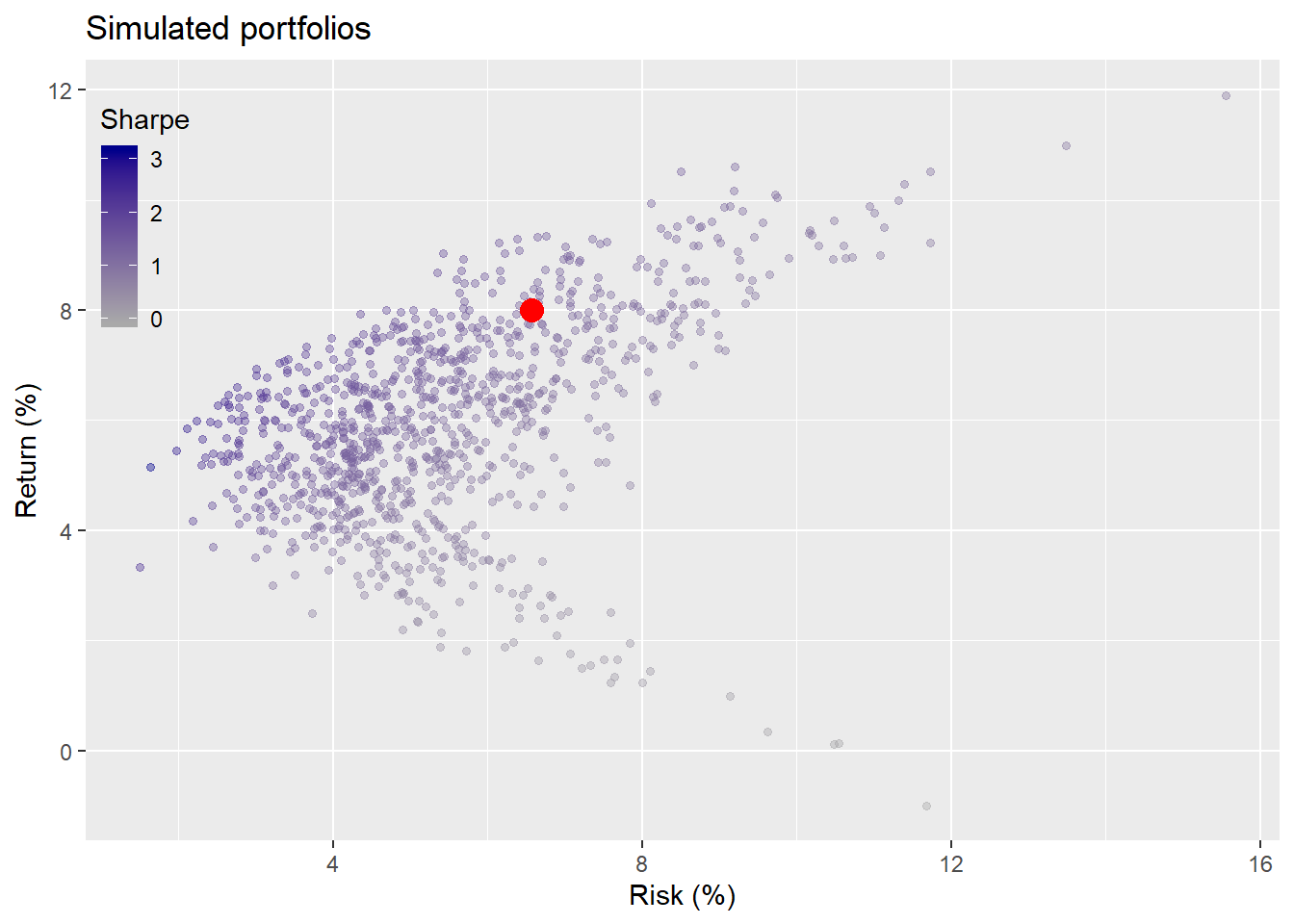As is clear, the portfolio ends up in the higher end of the continuum, but there are other portfolios that dominate it. Now the moment we’ve been waiting for—portfolio optimization! Taking a range of returns between the minimum and maximum of the simulated portfolios, we’ll calculate the optimal weights to produce the highest return for the lowest amount of risk.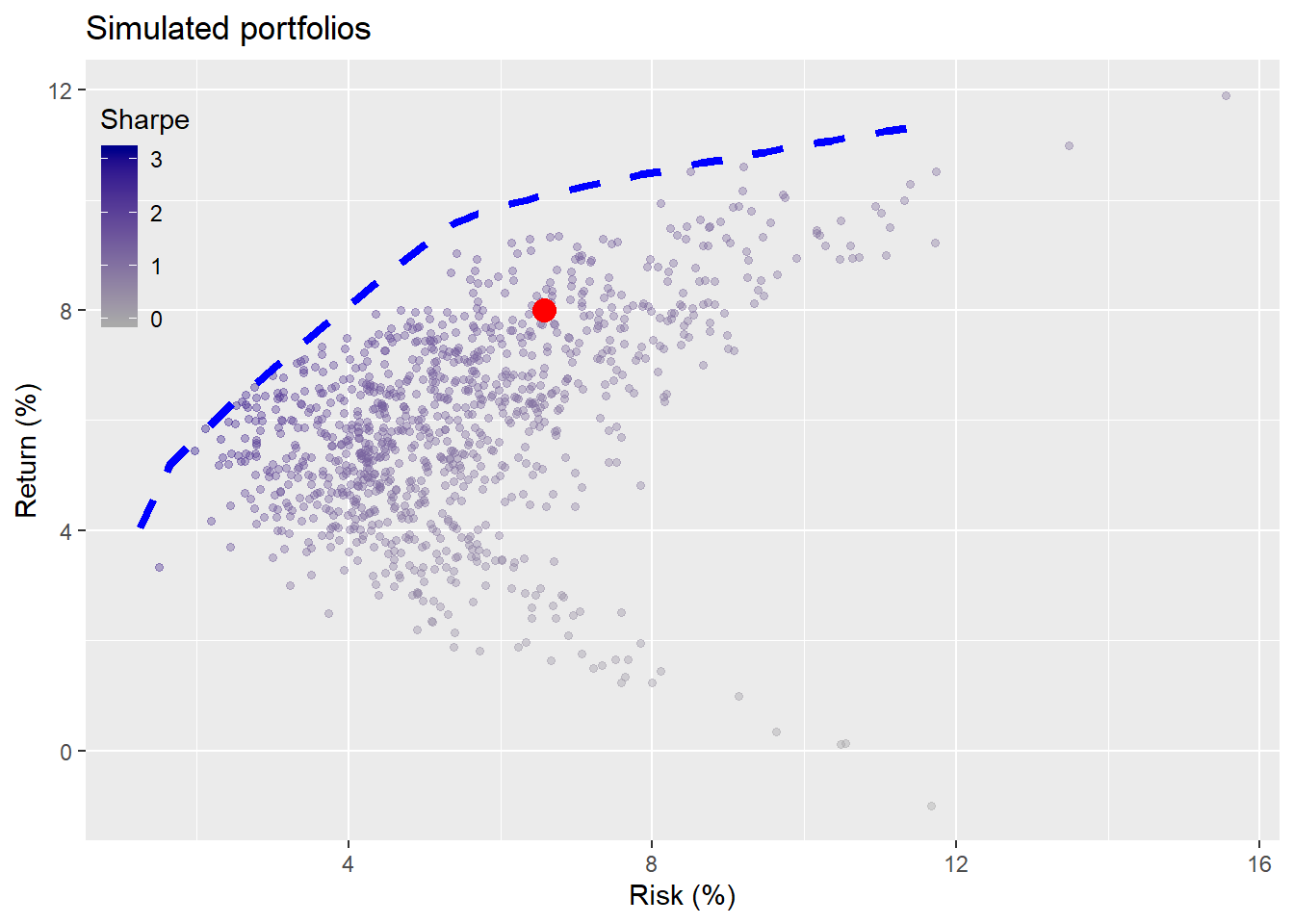Wow! That optimization stuff sure does work. The blue line representing the efficient frontier clearly shows that there are other portfolios that could generate much higher returns for the implied level of risk we’re taking on. Alternatively, if we move horizontally to the left we see that we could achieve the same level of return at a much lower level of risk, shown by where the blue line crosses above 7% return.

Recall for illustrative purposes we used a simple version for the original weight simulation that required an investment in all assets. When we relax that constraint, we get a much wider range of outcomes, as we pointed out in the last post. What if we ran the weighting simulation with the relaxed constraint? What would our simulation and allocation look like in that case? We show those results below.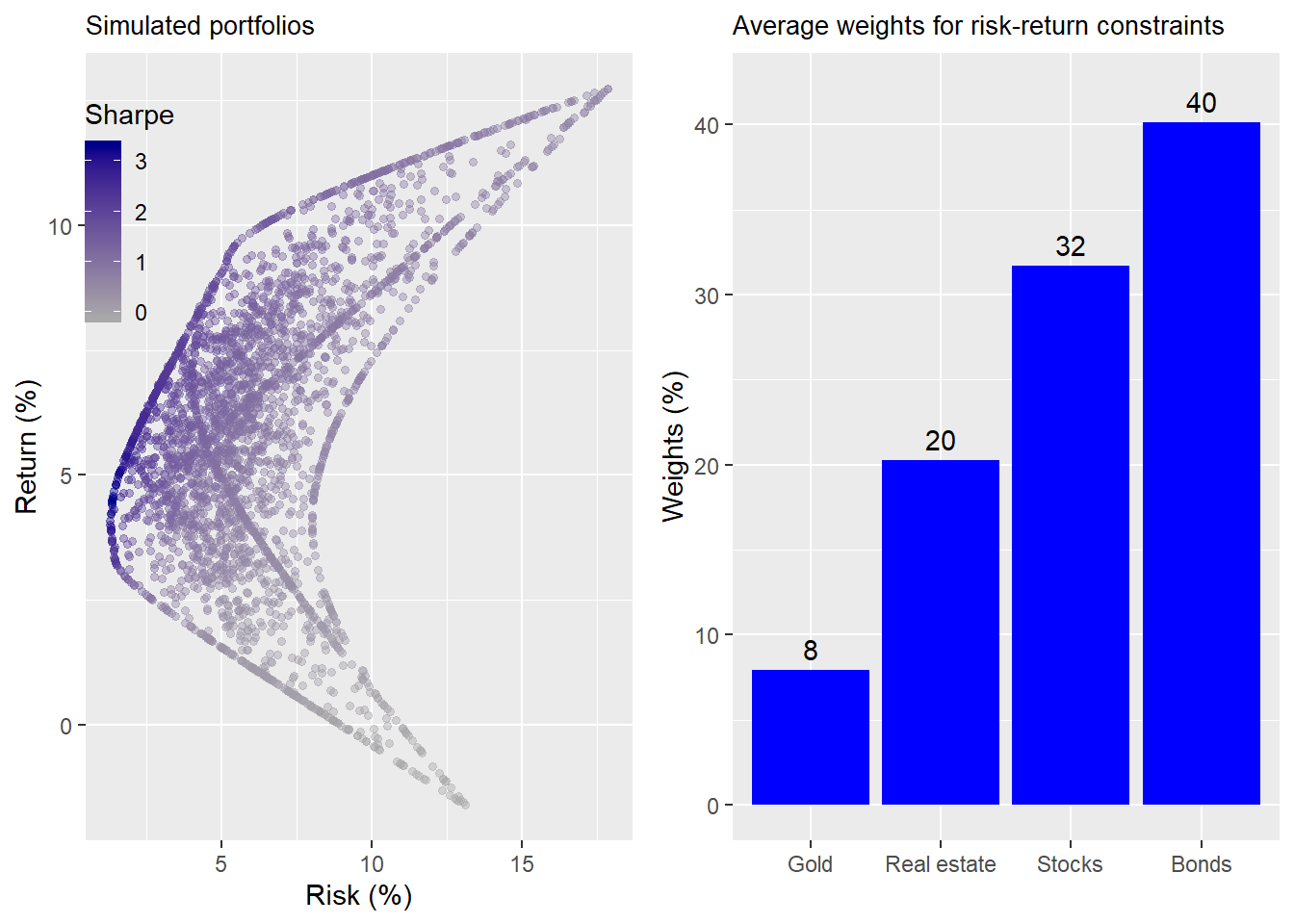We see a much broader range of outcomes, which yields a higher weighting to bonds and a lower one to gold than the previous portfolio. Now we’ll overlay the placement of our satisfactory portfolio on the broader weight simulation along with the efficient frontier in the graph below.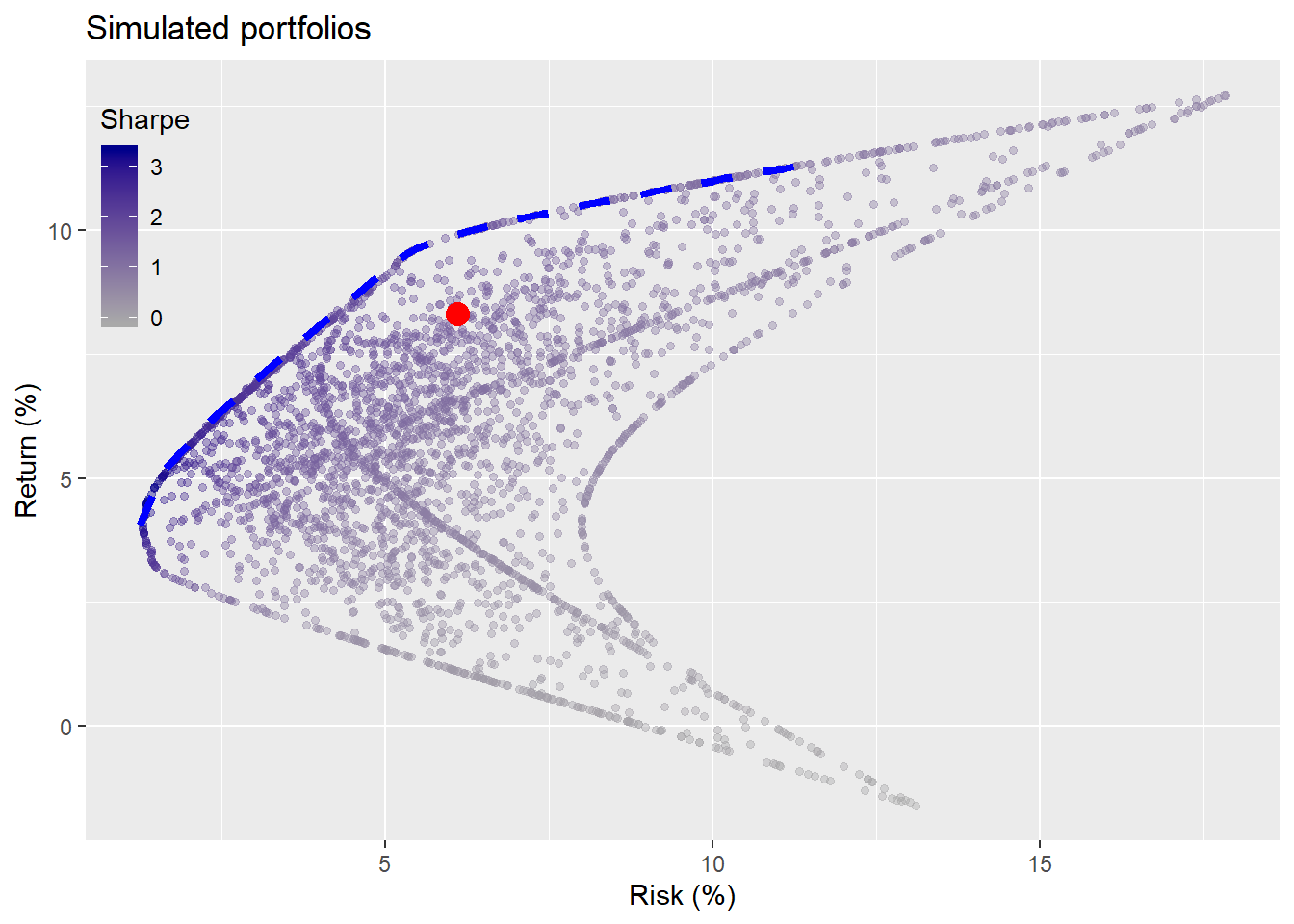Who needs mean-variance optimization when you’ve got data science simulation?! As one can see, when you allow portfolio weights to approach zero in many, but not all, of the assets, you can approximate the efficient frontier without having to rely on quadratic programming. This should give new meaning to “p-hacking.” Still, quadratic programming is likely to be a lot faster that running thousands of simulations with a large portfolio of assets. Recall for the four asset portfolio when we relaxed the inclusion constraint, that tripled the number of simulations. Hence, for any simulation in which some portfolios won’t be invested in all the assets, the number of calculations increases by a factor of the total number of assets minus one.

Whatever the case, we see that the satisfactory portfolio may not be that satisfactory given how much it’s dominated by the efficient frontier. Recall, however, we weren’t trying to achieve an optimal portfolio per se. We “just” wanted a portfolio that would meet our risk-return constraints.

Let’s see what happens when we use our satisfactory portfolio’s weights on the first five-year test period. In the graph below, we calculate our portfolios risk and return and then place it within our weight simulation scatter plot. We also calculate the risk and returns of various portfolios using the weights we derived from our efficient frontier above and add that our graph as the blue line.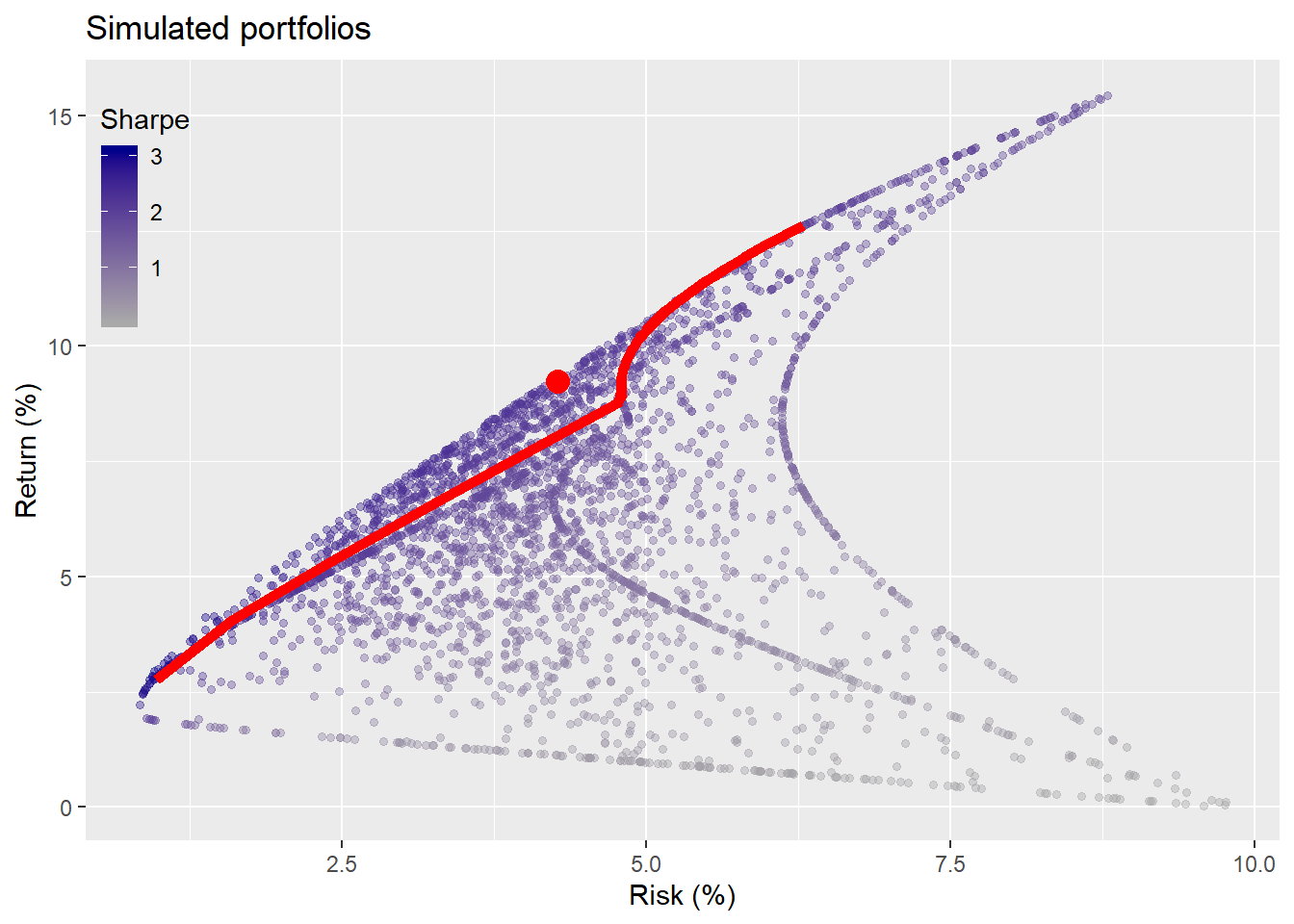Uh oh, not so efficient. The weights from the previous efficient frontier did not achieve optimal portfolios in the future and produced an unusual shape too. This illustrates one of the main problems with mean-variance optimization: “optimal weights are sensitive to return estimates”. In other words, if your estimate of returns aren’t that great, your optimal portfolio weights won’t be so optimal. Moreover, even if your estimates reflect all presently available information, that doesn’t mean they’ll be that accurate in the future.

A great way to see this is to calculate the efficient frontier using as much of the data as we have, ignoring incomplete cases (which produces bias) and plotting that against the original and first five-year simulations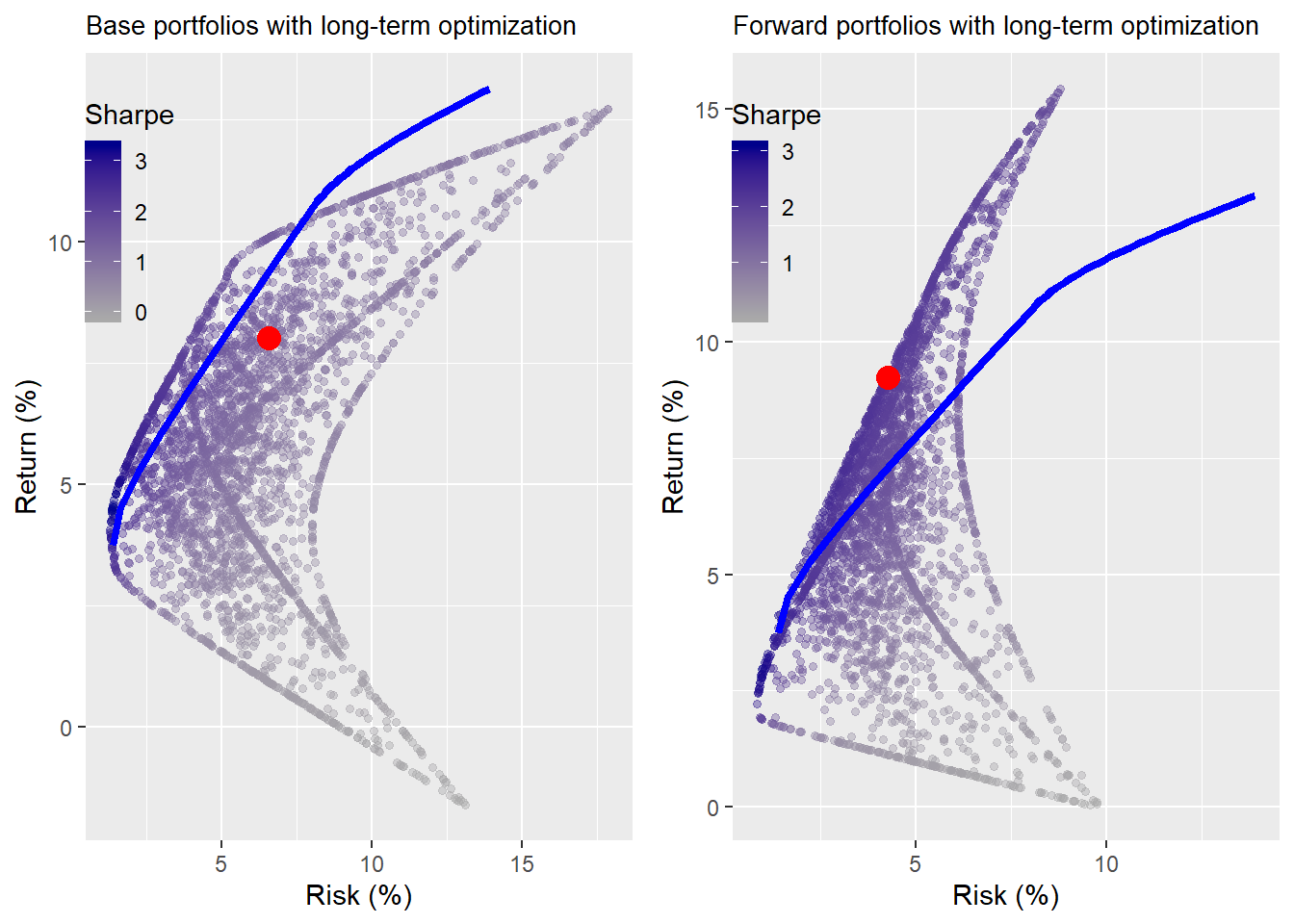You win some; you lose some. As is evident, different return estimates yield different frontiers both retrospectively and prospectively. Should we be skeptical of mean mean-variance optimization as Warren Buffett is of “geeks bearing gifts”? Not really. It’s an elegant solution to the thorny problem of portfolio construction. But it’s not very dynamic and it doesn’t exactly allow for much uncertainty around estimates.

There have been a number attempts to address such shortcomings including multi-period models, inter-temporal models, and even a statistics-free approach, among others. Even summarizing these different approaches would take us far afield of this post. Suffice it to say, there isn’t a clear winner; instead each refinement addresses a particular issue or fits a particular risk preference.

We’ve now partially revealed why we’ve been talking about a “satisfactory” portfolio all along. It’s the trade-off between satsificing and optimal. While we cannot possibly discuss all the nuances of satisficing now, our brief explanation is this. Satisficing is finding the best available solution when the optimal one is uncertain or unattainable. It was a concept developed by Herbert Simon who argued that decision makers could choose an optimal solution to a simplified reality or a satisfactory solution to a messy one.

If the “optimal” solution to portfolio allocation is a moving target with multiple approaches to calculating it, many of which involve a great deal of complexity, then electing a “good-enough” solution might be more satisfactory. The cost to become conversant in the technical details necessary to understand some of the solutions, let alone compile all the data necessary, could be prohibitive. Of course, if you’re a fund manager being paid to outperform (i.e., beat everyone else trying to beat you), then it behooves you to seek out these arcane solutions if your commpetitors are apt to use them too.

This discussion explains, in part, why the “simple” 1/n or 60/40 stock/bond portfolios are so popular. The exercise of mean-variance optimization and all its offshoots may simply be too much effort if the answers it gives aren’t dramatically better than a simplified approach. But it would be wrong to lay the blame for poor results or uncertainty on MVO: financial markets have way more noise than signal.

In pursuit of the signal, our next posts will look at the “simple” portfolios and see what they produce over multiple simulations relative to the satisfactory and optimal portfolios we’ve already discussed. If you think this blog is producing more noise than signal or vice versa, we want to know! Our email address is after the R and Python code below.

R code:

# Written in R 3.6.2
# Code for any source('function.R') is found at the end.

suppressPackageStartupMessages({
library(tidyquant)
library(tidyverse)
})

sym_names <- c("stock", "bond", "gold", "realt", "rfr")

## Call simuation functions
source("Portfolio_simulation_functions.R")

## Run simulation
set.seed(123)
port_sim_1 <- port_sim(df[2:61,2:5],1000,4)

## Graph
port_sim_1$graph + theme(legend.position = c(0.05,0.8), legend.key.size = unit(.5, "cm"), legend.background = element_rect(fill = NA)) ## Run selection function and graph results_1 <- port_select_func(port_sim_1, 0.07, 0.1, sym_names[1:4]) results_1$graph

# Create satisfactory portfolio
satis_ret <- sum(results_1$port_wts*colMeans(df[2:61, 2:5])) satis_risk <- sqrt(as.numeric(results_1$port_wts) %*%
cov(df[2:61, 2:5]) %*% as.numeric(results_1$port_wts)) port_satis <- data.frame(returns = satis_ret, risk = satis_risk) # Graph with simulated port_sim_1$graph +
geom_point(data = port_satis,
aes(risk*sqrt(12)*100, returns*1200),
size = 4,
color="red") +
theme(legend.position = c(0.05,0.8), legend.key.size = unit(.5, "cm"),
legend.background = element_rect(fill = NA))

## Find efficient frontier
source("Efficient_frontier.R")
eff_port <- eff_frontier_long(df[2:61,2:5], risk_increment = 0.01)

df_eff <- data.frame(returns = eff_port$exp_ret, risk = eff_port$stdev)

port_sim_1$graph + geom_line(data = df_eff, aes(risk*sqrt(12)*100, returns*1200), color = 'blue', size = 1.5, linetype = "dashed") + geom_point(data = port_satis, aes(risk*sqrt(12)*100, returns*1200), size = 4, color="red") + theme(legend.position = c(0.05,0.8), legend.key.size = unit(.5, "cm"), legend.background = element_rect(fill = NA)) # Simulation with leaving out assets port_sim_1lv <- port_sim_lv(df[2:61,2:5],1000,4) lv_graf <- port_sim_1lv$graph +
theme(legend.position = c(0.05,0.8), legend.key.size = unit(.5, "cm"),
legend.background = element_rect(fill = NA),
plot.title = element_text(size=10))

## Run selection function
results_1lv <- port_select_func(port_sim_1lv, 0.07, 0.1, sym_names[1:4])
lv_res_graf <- results_1lv$graph + theme(plot.title = element_text(size=10)) gridExtra::grid.arrange(lv_graf, lv_res_graf, ncol=2) ## Create satisfactory data frame and graph leave out portfolios with efficient frontier satis_ret_lv <- sum(results_1lv$port_wts*colMeans(df[2:61, 2:5]))
satis_risk_lv <- sqrt(as.numeric(results_1lv$port_wts) %*% cov(df[2:61, 2:5]) %*% as.numeric(results_1lv$port_wts))

port_satis_lv <- data.frame(returns = satis_ret_lv, risk = satis_risk_lv)

port_sim_1lv$graph + geom_line(data = df_eff, aes(risk*sqrt(12)*100, returns*1200), color = 'blue', size = 1.5, linetype = "dashed") + geom_point(data = port_satis_lv, aes(risk*sqrt(12)*100, returns*1200), size = 4, color="red") + theme(legend.position = c(0.05,0.8), legend.key.size = unit(.5, "cm"), legend.background = element_rect(fill = NA)) ## Run function and create actual portfolio and data frame for graph port_1_act <- rebal_func(df[62:121,2:5],results_1lv$port_wts)

port_act <- data.frame(returns = mean(port_1_act$ret_vec), risk = sd(port_1_act$ret_vec),
sharpe = mean(port_1_act$ret_vec)/sd(port_1_act$ret_vec)*sqrt(12))

## Simulate portfolios on first five-year period
set.seed(123)
port_sim_2 <- port_sim_lv(df[62:121,2:5], 1000, 4)

eff_ret1 <- apply(eff_port[,1:4], 1, function(x) x %*% colMeans(df[62:121, 2:5]))
eff_risk1 <- sqrt(apply(eff_port[,1:4],
1,
function(x)
as.numeric(x) %*% cov(df[62:121,2:5]) %*% as.numeric(x)))

eff_port1 <- data.frame(returns = eff_ret1, risk = eff_risk1)

## Graph simulation with chosen portfolio
port_sim_2$graph + geom_point(data = port_act, aes(risk*sqrt(12)*100, returns*1200), size = 4, color="red") + geom_line(data = eff_port1, aes(risk*sqrt(12)*100, returns*1200), color = 'red', size = 2) + theme(legend.position = c(0.05,0.8), legend.key.size = unit(.5, "cm"), legend.background = element_rect(fill = NA)) ## Using longer term data eff_port_old <- eff_frontier_long(dat[1:253,2:5], risk_increment = 0.01) df_eff_old <- data.frame(returns = eff_port_old$exp_ret, risk = eff_port_old$stdev) p1 <- port_sim_1lv$graph +
geom_line(data = df_eff_old,
aes(risk*sqrt(12)*100, returns*1200),
color = 'blue',
size = 1.5) +
geom_point(data = port_satis,
aes(risk*sqrt(12)*100, returns*1200),
size = 4,
color="red") +
theme(legend.position = c(0.05,0.8), legend.key.size = unit(.5, "cm"),
legend.background = element_rect(fill = NA),
plot.title = element_text(size=10)) +
labs(title = 'Simulated portfolios with long-term optimzation')

# For forward graph
eff_ret1_old <- apply(eff_port_old[,1:4], 1,
function(x) x %*% colMeans(dat[1:253, 2:5], na.rm = TRUE))
eff_risk1_old <- sqrt(apply(eff_port_old[,1:4],
1,
function(x)
as.numeric(x) %*%
cov(dat[1:253,2:5],
use = 'pairwise.complete.obs') %*%
as.numeric(x)))

eff_port1_old <- data.frame(returns = eff_ret1_old, risk = eff_risk1_old)

## Graph simulation with chosen portfolio
p2 <- port_sim_2$graph + geom_point(data = port_act, aes(risk*sqrt(12)*100, returns*1200), size = 4, color="red") + geom_line(data = eff_port1_old, aes(risk*sqrt(12)*100, returns*1200), color = 'blue', size = 2) + theme(legend.position = c(0.05,0.8), legend.key.size = unit(.5, "cm"), legend.background = element_rect(fill = NA), plot.title = element_text(size=10)) + labs(title = 'Forward portfolios with long-term optimization') gridExtra::grid.arrange(p1, p2, ncol=2) #### Portfolio_simulation_functions.R # Portfolio simulations ## Portfolio simuation function port_sim <- function(df, sims, cols){ if(ncol(df) != cols){ print("Columns don't match") break } # Create weight matrix wts <- matrix(nrow = sims, ncol = cols) for(i in 1:sims){ a <- runif(cols,0,1) b <- a/sum(a) wts[i,] <- b } # Find returns mean_ret <- colMeans(df) # Calculate covariance matrix cov_mat <- cov(df) # Calculate random portfolios port <- matrix(nrow = sims, ncol = 2) for(i in 1:sims){ port[i,1] <- as.numeric(sum(wts[i,] * mean_ret)) port[i,2] <- as.numeric(sqrt(t(wts[i,]) %*% cov_mat %*% wts[i,])) } colnames(port) <- c("returns", "risk") port <- as.data.frame(port) port$Sharpe <- port$returns/port$risk*sqrt(12)

max_sharpe <- port[which.max(port$Sharpe),] graph <- port %>% ggplot(aes(risk*sqrt(12)*100, returns*1200, color = Sharpe)) + geom_point(size = 1.2, alpha = 0.4) + scale_color_gradient(low = "darkgrey", high = "darkblue") + labs(x = "Risk (%)", y = "Return (%)", title = "Simulated portfolios") out <- list(port = port, graph = graph, max_sharpe = max_sharpe, wts = wts) } ## Portfolio Simulation leave port_sim_lv <- function(df, sims, cols){ if(ncol(df) != cols){ print("Columns don't match") break } # Create weight matrix wts <- matrix(nrow = (cols-1)*sims, ncol = cols) count <- 1 for(i in 1:(cols-1)){ for(j in 1:sims){ a <- runif((cols-i+1),0,1) b <- a/sum(a) c <- sample(c(b,rep(0,i-1))) wts[count,] <- c count <- count+1 } } # Find returns mean_ret <- colMeans(df) # Calculate covariance matrix cov_mat <- cov(df) # Calculate random portfolios port <- matrix(nrow = (cols-1)*sims, ncol = 2) for(i in 1:nrow(port)){ port[i,1] <- as.numeric(sum(wts[i,] * mean_ret)) port[i,2] <- as.numeric(sqrt(t(wts[i,]) %*% cov_mat %*% wts[i,])) } colnames(port) <- c("returns", "risk") port <- as.data.frame(port) port$Sharpe <- port$returns/port$risk*sqrt(12)

max_sharpe <- port[which.max(port$Sharpe),] graph <- port %>% ggplot(aes(risk*sqrt(12)*100, returns*1200, color = Sharpe)) + geom_point(size = 1.2, alpha = 0.4) + scale_color_gradient(low = "darkgrey", high = "darkblue") + labs(x = "Risk (%)", y = "Return (%)", title = "Simulated portfolios") out <- list(port = port, graph = graph, max_sharpe = max_sharpe, wts = wts) } ## Load portfolio selection function port_select_func <- function(port, return_min, risk_max, port_names){ port_select <- cbind(port$port, port$wts) port_wts <- port_select %>% mutate(returns = returns*12, risk = risk*sqrt(12)) %>% filter(returns >= return_min, risk <= risk_max) %>% summarise_at(vars(4:7), mean) %>% colnames<-(port_names) p <- port_wts %>% rename("Stocks" = stock, "Bonds" = bond, "Gold" = gold, "Real estate" = realt) %>% gather(key,value) %>% ggplot(aes(reorder(key,value), value*100 )) + geom_bar(stat='identity', position = "dodge", fill = "blue") + geom_text(aes(label=round(value,2)*100), vjust = -0.5) + scale_y_continuous(limits = c(0,max(port_wts*100+2))) + labs(x="", y = "Weights (%)", title = "Average weights for risk-return constraints") out <- list(port_wts = port_wts, graph = p) out } ## Function for portfolio returns without rebalancing rebal_func <- function(act_ret, weights){ ret_vec <- c() wt_mat <- matrix(nrow = nrow(act_ret), ncol = ncol(act_ret)) for(i in 1:nrow(wt_mat)){ wt_ret <- act_ret[i,]*weights # wt'd return ret <- sum(wt_ret) # total return ret_vec[i] <- ret weights <- (weights + wt_ret)/(sum(weights)+ret) # new weight based on change in asset value wt_mat[i,] <- as.numeric(weights) } out <- list(ret_vec = ret_vec, wt_mat = wt_mat) out } #### Efficient_frontier.R # Adapted from https://www.nexteinstein.org/wp-content/uploads/sites/6/2017/01/ORIG_Portfolio-Optimization-Using-R_Pseudo-Code.pdf eff_frontier_long <- function(returns, risk_premium_up = 0.5, risk_increment = 0.005){ covariance <- cov(returns, use = "pairwise.complete.obs") num <- ncol(covariance) Amat <- cbind(1, diag(num)) bvec <- c(1, rep(0, num)) meq <- 1 risk_steps <- risk_premium_up/risk_increment+1 count <- 1 eff <- matrix(nrow = risk_steps, ncol = num + 3) colnames(eff) <- c(colnames(returns), "stdev", "exp_ret", "sharpe") loop_step <- seq(0, risk_premium_up, risk_increment) for(i in loop_step){ dvec <- colMeans(returns, na.rm = TRUE)*i sol <- quadprog::solve.QP(covariance, dvec = dvec, Amat = Amat, bvec = bvec, meq = meq) eff[count, "stdev"] <- sqrt(sum(sol$solution * colSums(covariance * sol$solution))) eff[count, "exp_ret"] <- as.numeric(sol$solution %*% colMeans(returns, na.rm = TRUE))
eff[count, "sharpe"] <- eff[count,"exp_ret"]/eff[count, "stdev"]
eff[count, 1:num] <- sol\$solution
count <- count + 1
}
return(as.data.frame(eff))

}

Python code:

# Load libraries
import pandas as pd
import numpy as np
import matplotlib.pyplot as plt
%matplotlib inline

plt.style.use('ggplot')

# SKIP IF ALREADY HAVE DATA
start_date = '1970-01-01'
end_date = '2019-12-31'
symbols = ["WILL5000INDFC", "BAMLCC0A0CMTRIV", "GOLDPMGBD228NLBM", "CSUSHPINSA", "DGS5"]
sym_names = ["stock", "bond", "gold", "realt", 'rfr']
filename = 'data_port_const.pkl'

try:
except FileNotFoundError:
data = web.DataReader(symbols, 'fred', start_date, end_date)
data.columns = sym_names

data_mon = data.resample('M').last()
df = data_mon.pct_change()['1987':'2019']
# df.to_pickle(filename) # If you haven't saved the file

dat = data_mon.pct_change()['1971':'2019']
# pd.to_pickle(df,filename) # if you haven't saved the file

# Portfolio simulation functions

## Simulation function
class Port_sim:
def calc_sim(df, sims, cols):
wts = np.zeros((sims, cols))

for i in range(sims):
a = np.random.uniform(0,1,cols)
b = a/np.sum(a)
wts[i,] = b

mean_ret = df.mean()
port_cov = df.cov()

port = np.zeros((sims, 2))
for i in range(sims):
port[i,0] =  np.sum(wts[i,]*mean_ret)
port[i,1] = np.sqrt(np.dot(np.dot(wts[i,].T,port_cov), wts[i,]))

sharpe = port[:,0]/port[:,1]*np.sqrt(12)
best_port = port[np.where(sharpe == max(sharpe))]
max_sharpe = max(sharpe)

return port, wts, best_port, sharpe, max_sharpe

def calc_sim_lv(df, sims, cols):
wts = np.zeros(((cols-1)*sims, cols))
count=0

for i in range(1,cols):
for j in range(sims):
a = np.random.uniform(0,1,(cols-i+1))
b = a/np.sum(a)
c = np.random.choice(np.concatenate((b, np.zeros(i))),cols, replace=False)
wts[count,] = c
count+=1

mean_ret = df.mean()
port_cov = df.cov()

port = np.zeros(((cols-1)*sims, 2))
for i in range(sims):
port[i,0] =  np.sum(wts[i,]*mean_ret)
port[i,1] = np.sqrt(np.dot(np.dot(wts[i,].T,port_cov), wts[i,]))

sharpe = port[:,0]/port[:,1]*np.sqrt(12)
best_port = port[np.where(sharpe == max(sharpe))]
max_sharpe = max(sharpe)

return port, wts, best_port, sharpe, max_sharpe

def graph_sim(port, sharpe):
plt.figure(figsize=(14,6))
plt.scatter(port[:,1]*np.sqrt(12)*100, port[:,0]*1200, marker='.', c=sharpe, cmap='Blues')
plt.colorbar(label='Sharpe ratio', orientation = 'vertical', shrink = 0.25)
plt.title('Simulated portfolios', fontsize=20)
plt.xlabel('Risk (%)')
plt.ylabel('Return (%)')
plt.show()

# Constraint function
def port_select_func(port, wts, return_min, risk_max):
port_select = pd.DataFrame(np.concatenate((port, wts), axis=1))
port_select.columns = ['returns', 'risk', 1, 2, 3, 4]

port_wts = port_select[(port_select['returns']*12 >= return_min) & (port_select['risk']*np.sqrt(12) <= risk_max)]
port_wts = port_wts.iloc[:,2:6]
port_wts = port_wts.mean(axis=0)
return port_wts

def port_select_graph(port_wts):
plt.figure(figsize=(12,6))
key_names = {1:"Stocks", 2:"Bonds", 3:"Gold", 4:"Real estate"}
lab_names = []
graf_wts = port_wts.sort_values()*100

for i in range(len(graf_wts)):
name = key_names[graf_wts.index[i]]
lab_names.append(name)

plt.bar(lab_names, graf_wts, color='blue')
plt.ylabel("Weight (%)")
plt.title("Average weights for risk-return constraint", fontsize=15)

for i in range(len(graf_wts)):
plt.annotate(str(round(graf_wts.values[i])), xy=(lab_names[i], graf_wts.values[i]+0.5))

plt.show()

# Return function with no rebalancing
def rebal_func(act_ret, weights):
ret_vec = np.zeros(len(act_ret))
wt_mat = np.zeros((len(act_ret), len(act_ret.columns)))
for i in range(len(act_ret)):
wt_ret = act_ret.iloc[i,:].values*weights
ret = np.sum(wt_ret)
ret_vec[i] = ret
weights = (weights + wt_ret)/(np.sum(weights) + ret)
wt_mat[i,] = weights

return ret_vec, wt_mat

## Rum simulation and graph
np.random.seed(123)
port_sim_1, wts_1, _, sharpe_1, _ = Port_sim.calc_sim(df.iloc[1:60,0:4],1000,4)

Port_sim.graph_sim(port_sim_1, sharpe_1)

# Weight choice
results_1_wts = port_select_func(port_sim_1, wts_1, 0.07, 0.1)
port_select_graph(results_1_wts)

# Compute satisfactory portfolio
satis_ret = np.sum(results_1_wts * df.iloc[1:60,0:4].mean(axis=0).values)
satis_risk = np.sqrt(np.dot(np.dot(results_1_wts.T, df.iloc[1:60,0:4].cov()),results_1_wts))

# Graph simulation with actual portfolio return
plt.figure(figsize=(14,6))
plt.scatter(port_sim_1[:,1]*np.sqrt(12)*100, port_sim_1[:,0]*1200, marker='.', c=sharpe_1, cmap='Blues')
plt.colorbar(label='Sharpe ratio', orientation = 'vertical', shrink = 0.25)
plt.scatter(satis_risk*np.sqrt(12)*100, satis_ret*1200, c='red', s=50)
plt.title('Simulated portfolios', fontsize=20)
plt.xlabel('Risk (%)')
plt.ylabel('Return (%)')
plt.show()

# Create efficient frontier function
from scipy.optimize import minimize

def eff_frontier(df_returns, min_ret, max_ret):

n = len(df_returns.columns)

def get_data(weights):
weights = np.array(weights)
returns = np.sum(df_returns.mean() * weights)
risk = np.sqrt(np.dot(weights.T, np.dot(df_returns.cov(), weights)))
sharpe = returns/risk
return np.array([returns,risk,sharpe])

# Contraints
def check_sum(weights):
return np.sum(weights) - 1

# Rante of returns
mus = np.linspace(min_ret,max_ret,20)

# Function to minimize
def minimize_volatility(weights):
return  get_data(weights)

# Inputs
init_guess = np.repeat(1/n,n)
bounds = tuple([(0,1) for _ in range(n)])

eff_risk = []
port_weights = []

for mu in mus:
# function for return
cons = ({'type':'eq','fun': check_sum},
{'type':'eq','fun': lambda w: get_data(w) - mu})

result = minimize(minimize_volatility,init_guess,method='SLSQP',bounds=bounds,constraints=cons)

eff_risk.append(result['fun'])
port_weights.append(result.x)

eff_risk = np.array(eff_risk)

return mus, eff_risk, port_weights

## Create variables for froniter function
df_returns = df.iloc[1:60, 0:4]
min_ret = min(port_sim_1[:,0])
max_ret = max(port_sim_1[:,0])

eff_ret, eff_risk, eff_weights = eff_frontier(df_returns, min_ret, max_ret)

## Graph efficient frontier

plt.figure(figsize=(12,6))
plt.scatter(port_sim_1[:,1]*np.sqrt(12)*100, port_sim_1[:,0]*1200, marker='.', c=sharpe_1, cmap='Blues')
plt.plot(eff_risk*np.sqrt(12)*100,eff_ret*1200,'b--',linewidth=2)
plt.scatter(satis_risk*np.sqrt(12)*100, satis_ret*1200, c='red', s=50)

plt.colorbar(label='Sharpe ratio', orientation = 'vertical', shrink = 0.25)
plt.title('Simulated portfolios', fontsize=20)
plt.xlabel('Risk (%)')
plt.ylabel('Return (%)')
plt.show()

## Graph with unconstrained weights
np.random.seed(123)
port_sim_1lv, wts_1lv, _, sharpe_1lv, _ = Port_sim.calc_sim_lv(df.iloc[1:60,0:4],1000,4)

Port_sim.graph_sim(port_sim_1lv, sharpe_1lv)

# Weight choice
results_1lv_wts = port_select_func(port_sim_1lv, wts_1lv, 0.07, 0.1)
port_select_graph(results_1lv_wts)

# Satisfactory portfolio unconstrained weights
satis_ret1 = np.sum(results_1lv_wts * df.iloc[1:60,0:4].mean(axis=0).values)
satis_risk1 = np.sqrt(np.dot(np.dot(results_1lv_wts.T, df.iloc[1:60,0:4].cov()),results_1lv_wts))

# Graph with efficient frontier
plt.figure(figsize=(12,6))
plt.scatter(port_sim_1lv[:,1]*np.sqrt(12)*100, port_sim_1lv[:,0]*1200, marker='.', c=sharpe_1lv, cmap='Blues')
plt.plot(eff_risk*np.sqrt(12)*100,eff_ret*1200,'b--',linewidth=2)
plt.scatter(satis_risk1*np.sqrt(12)*100, satis_ret1*1200, c='red', s=50)

plt.colorbar(label='Sharpe ratio', orientation = 'vertical', shrink = 0.25)
plt.title('Simulated portfolios', fontsize=20)
plt.xlabel('Risk (%)')
plt.ylabel('Return (%)')
plt.show()

# Five year forward with unconstrained satisfactory portfolio
# Returns
## Run rebalance function using desired weights
port_1_act, wt_mat = rebal_func(df.iloc[61:121,0:4], results_1lv_wts)
port_act = {'returns': np.mean(port_1_act),
'risk': np.std(port_1_act),
'sharpe': np.mean(port_1_act)/np.std(port_1_act)*np.sqrt(12)}

# Run simulation on recent five-years
np.random.seed(123)
port_sim_2lv, wts_2lv, _, sharpe_2lv, _ = Port_sim.calc_sim_lv(df.iloc[61:121,0:4],1000,4)

# Graph simulation with actual portfolio return
plt.figure(figsize=(14,6))
plt.scatter(port_sim_2lv[:,1]*np.sqrt(12)*100, port_sim_2lv[:,0]*1200, marker='.', c=sharpe_2lv, cmap='Blues')
plt.plot(eff_risk*np.sqrt(12)*100,eff_ret*1200,'b--',linewidth=2)
plt.scatter(port_act['risk']*np.sqrt(12)*100, port_act['returns']*1200, c='red', s=50)

plt.colorbar(label='Sharpe ratio', orientation = 'vertical', shrink = 0.25)
plt.title('Simulated portfolios', fontsize=20)
plt.xlabel('Risk (%)')
plt.ylabel('Return (%)')
plt.show()

## Eficient frontier on long term data
df_returns_l = dat.iloc[1:254, 0:4]
min_ret_l = min(port_sim_1[:,0])
max_ret_l = max(port_sim_1[:,0])

eff_ret_l, eff_risk_l, eff_weightsl = eff_frontier(df_returns1, min_ret1, max_ret1)

## Graph with original
plt.figure(figsize=(12,6))
plt.scatter(port_sim_1lv[:,1]*np.sqrt(12)*100, port_sim_1lv[:,0]*1200, marker='.', c=sharpe_1lv, cmap='Blues')
plt.plot(eff_risk_l*np.sqrt(12)*100,eff_ret_l*1200,'b--',linewidth=2)
plt.scatter(satis_risk1*np.sqrt(12)*100, satis_ret1*1200, c='red', s=50)

plt.colorbar(label='Sharpe ratio', orientation = 'vertical', shrink = 0.25)
plt.title('Simulated portfolios', fontsize=20)
plt.xlabel('Risk (%)')
plt.ylabel('Return (%)')
plt.show()

## Graph with five-year forward
# Graph simulation with actual portfolio return
plt.figure(figsize=(14,6))
plt.scatter(port_sim_2lv[:,1]*np.sqrt(12)*100, port_sim_2lv[:,0]*1200, marker='.', c=sharpe_2lv, cmap='Blues')
plt.plot(eff_risk_l*np.sqrt(12)*100,eff_ret_l*1200,'b--',linewidth=2)
plt.scatter(port_act['risk']*np.sqrt(12)*100, port_act['returns']*1200, c='red', s=50)

plt.colorbar(label='Sharpe ratio', orientation = 'vertical', shrink = 0.25)
plt.title('Simulated portfolios', fontsize=20)
plt.xlabel('Risk (%)')
plt.ylabel('Return (%)')
plt.show()

1. As opposed to linear since we’re dealing with squares

2. Which is often the historical average return